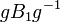# Borel-Morozov theorem

Jump to: navigation, search

## Statement

Suppose$G$ is a linear algebraic group over an algebraically closed field$K$. Then, the following are true:

1. Any two Borel subgroups of$G$ are conjugate subgroups.
2. Any closed connected solvable algebraic subgroup of$G$ is contained in a Borel subgroup.

## Related facts

### Facts with similar proofs

• Sylow implies order-dominating: This shows that any$p$-Sylow subgroup of a finite group contains some conjugate of any$p$-subgroup. It therefore show that both Sylow implis order-conjugate and that every$p$-subgroup is contained in some$p$-Sylow subgroup.

## Proof

We prove version (1). Version (2) is equivalent to it.

Given: A linear algebraic group$G$ over an algebraically closed field$K$. Borel subgroups$B_1, B_2$ of$G$.

To prove:$B_1$ is conjugate to$B_2$.

Proof:

Step no. Assertion/construction Facts used Given data used Previous steps used Explanation
1 The quotient variety$G/B_1$ is a complete algebraic variety. Fact (1)$B_1$ is Borel in$G$.
2$B_2$ is a connected solvable linear algebraic group over the algebraically closed field$K$.$B_2$ is a Borel subgroup of the linear algebraic group$G$$K$ is algebraically closed
follows from definition of Borel subgroup
3 Consider the action of$B_2$ on$G/B_1$ by left multiplication, obtained by restricting the action of$G$. This is an algebraic group action (i.e., it is regular in the algebraic group sense). The action is obtained by restricting a group multiplication, which is algebraic.
4 The action in Step (3) has a fixed point, say a coset$gB_1$. In other words,$B_2(gB_1) = gB_1$. Fact (2) Steps (1)-(3) Step-fact combination direct
5$B_2 \le gB_1g^{-1}$ Step (4) multiply both sides of the equality in step (4) on the right by$g^{-1}$, and get$B_2(gB_1g^{-1}) = gB_1g^{-1}$. Since both$B_2$ and$gB_1g^{-1}$ are subgroups, this gives the subgroup containment.
6$B_2 = gB_1g^{-1}$, so$B_1,B_2$ are conjugate subgroups.$B_1,B_2$ are Borel subgroups Step (5) Since$B_1$ is Borel, so is$gB_1g^{-1}$.$B_2$ is also Borel. Thus, both are maximal among connected closed solvable subgroups of$G$, and hence, by the containment of Step (5), they must be equal.